• ## 用python绘制散点图

万次阅读 多人点赞 2018-07-13 21:41:53
今天下午学习了如何使用python绘制简单的散点图，写成博客分享一下。 在python中画散点图主要是用matplotlib模块中的scatter函数，先来看一下scatter函数的基本信息。 网址为：点击打开链接 可以看到scatter中有很多...
在python中画散点图主要是用matplotlib模块中的scatter函数，先来看一下scatter函数的基本信息。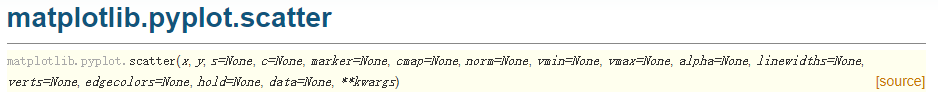网址为：点击打开链接

可以看到scatter中有很多参数，经常使用的参数主要有以下几个：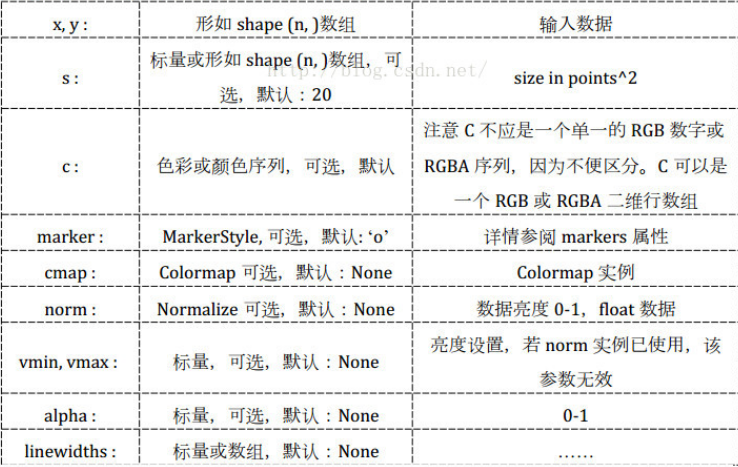c：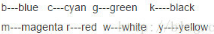marker: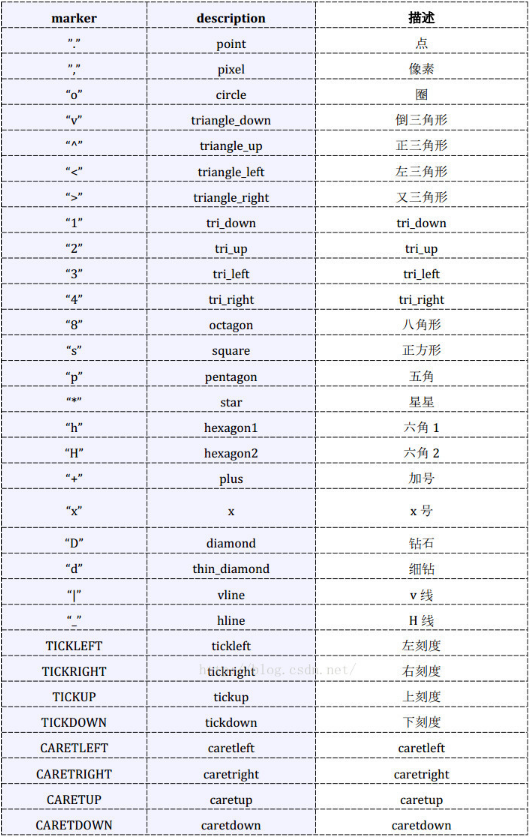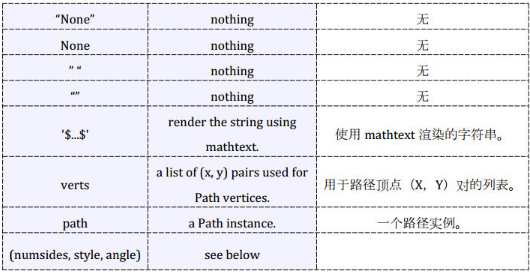数据、代码和绘制的图如下。

数据（取第一列作为x，取第四列作为y）截图：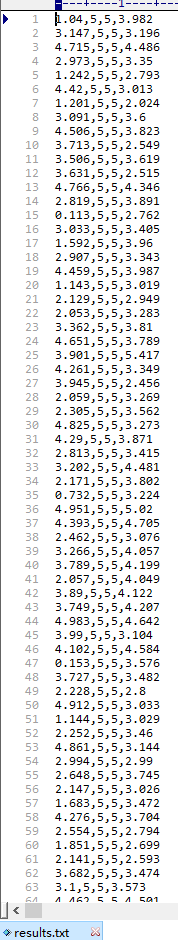代码如下：

import matplotlib.pyplot as plt
import numpy as np

# 定义画散点图的函数
def draw_scatter(n, s):
"""
:param n: 点的数量，整数
:param s:点的大小，整数
:return: None
"""
# 加载数据
# 通过切片获取横坐标x1
x1 = data[:, 0]
# 通过切片获取纵坐标R
y1 = data[:, 3]
# 横坐标x2
x2 = np.random.uniform(0, 5, n)
# 纵坐标y2
y2 = np.array( * n)
# 创建画图窗口
fig = plt.figure()
# 将画图窗口分成1行1列，选择第一块区域作子图
# 设置标题
ax1.set_title('Result Analysis')
# 设置横坐标名称
ax1.set_xlabel('gamma-value')
# 设置纵坐标名称
ax1.set_ylabel('R-value')
# 画散点图
ax1.scatter(x1, y1, s=s, c='k', marker='.')
# 画直线图
ax1.plot(x2, y2, c='b', ls='--')
# 调整横坐标的上下界
plt.xlim(xmax=5, xmin=0)
# 显示
plt.show()

# 主模块
if __name__ == "__main__":
# 运行
draw_scatter(n=2000, s=20)

绘制的图如下：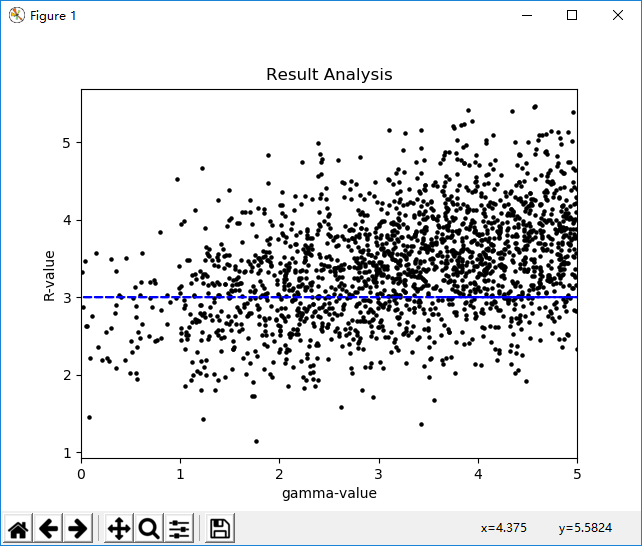展开全文• ## Python绘制折线图、散点图

万次阅读 多人点赞 2019-01-06 11:01:13
Python绘图需要下载安装matplotlib模块，它是一个数学绘图库，我们将使用它来制作简单的图表，如折线图散点图。关于matplotlib模块的下载安装预配置将在后面具体介绍。 （一）绘制折线图 首先，我们先绘制一个...Python绘图需要下载安装matplotlib模块，它是一个数学绘图库，我们将使用它来制作简单的图表，如折线图和散点图。关于matplotlib模块的下载安装预配置将在后面具体介绍。

（一）绘制折线图

首先，我们先绘制一个简单的折线图

# 调用模块

import matplotlib.pyplot as plt

squares=[1, 4, 9, 16, 25]
x=[1, 2, 3, 4, 5]
plt.plot(x, squares)

plt.show()

更多干货分享加python编程语言学习QQ群 515267276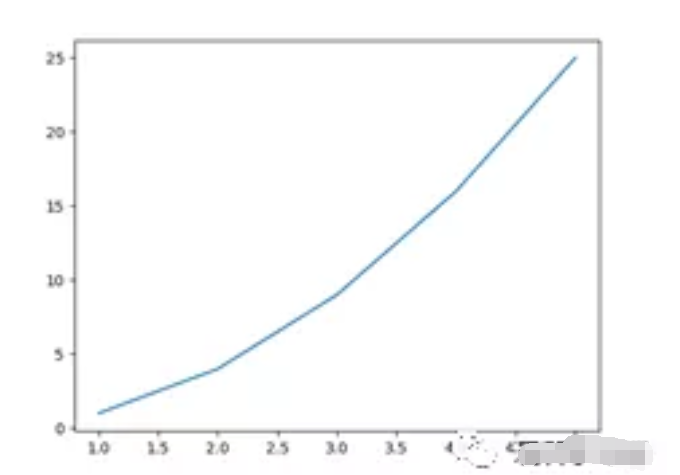解释：

（1）plt.plot(x, squares)作用是画图，其中x相当于横坐标轴，squaers相当于纵坐标轴；

（2）plt.show()作用是将画好的图显示出来。

接下来，对上图进行进一步的修饰。

import matplotlib.pyplot as plt
squares=[1, 4, 9, 16, 25]
x=[1, 2, 3, 4, 5]
# 设置线宽
plt.plot(x, squares, linewidth=4)

# 设置图表标题，并给坐标轴添加标签
plt.title("square of 'x'",

fontsize=20)
plt.xlabel("x", fontsize=12)
plt.ylabel("x^2", fontsize=12)

# 设置坐标轴刻度标记的大小
plt.tick_params(axis='both',

labelsize=10)
plt.show()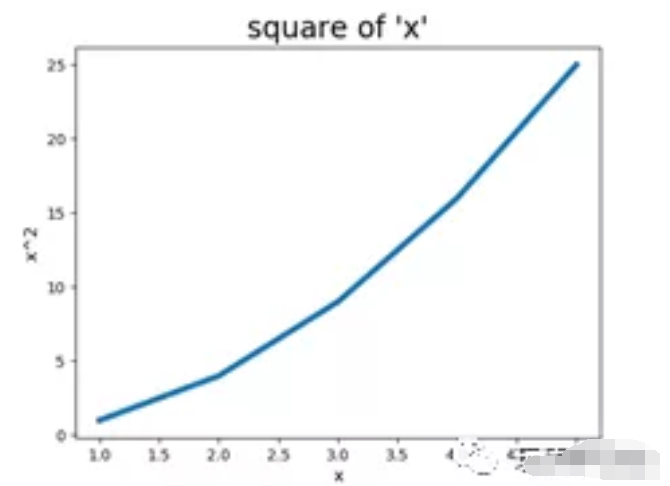解释：

（1）plt.plot(x, squares, linewidth=4)作用画图，参数linewidth=4表示将线条的宽度设置为4；

（2）plt.title("square of 'x'", fontsize=20)作用为图添加标题square of 'x'，参数fontsize表示设置字体的大小；

（3）plt.xlabel("x", fontsize=12)作用为横坐标轴添加标签’x’；

（4）plt.ylabel("x^2", fontsize=12)作用为纵坐标轴添加标签x^2；

（5）plt.tick_params(axis='both', labelsize=10)作用设置横纵坐标轴刻度的大小，此处大小为10。

（二）绘制散点图scatter

简单的画一个点

import matplotlib.pyplot as plt
plt.scatter(3, 9)
plt.show()

更多干货分享加python编程语言学习QQ群 515267276

画一些列点，并对画的图进行编辑修饰：

import matplotlib.pyplot as plt
x = [1, 2, 3, 4, 5]
squares = [1, 4, 9, 16, 25]

# 设置点的大小
plt.scatter(x, squares, s=200)

# 设置图表标题，并给坐标轴添加标签
plt.title("square of 'x'", fontsize=22)
plt.xlabel("x", fontsize=12)
plt.ylabel("x^2", fontsize=22)

# 设置坐标轴刻度标记的大小
plt.tick_params(axis='both',

which='major', labelsize=10)
plt.show()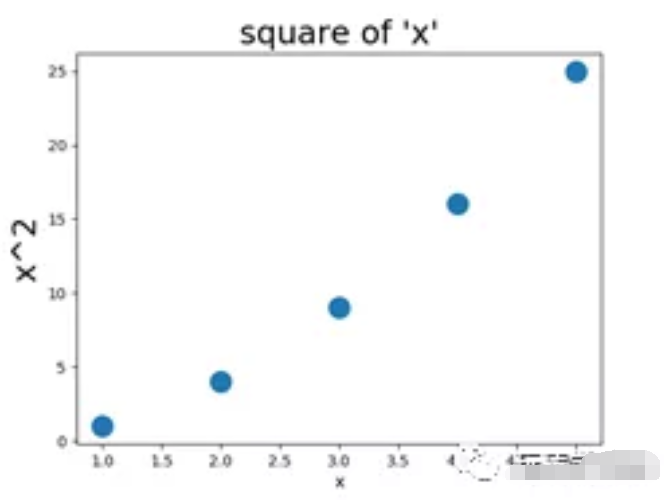可以自动计算数据：数值1到5与其对应的平方数的散点图：

import matplotlib.pyplot as plt
xlist = list(range(1,6))
ylist = [x**2 for x in xlist]
plt.scatter(xlist, ylist,

edgecolor='r', c='g', s=150)
plt.title("square of'x'", fontsize=22)
plt.xlabel("x", fontsize=22)
plt.ylabel("x^2", fontsize=22)
plt.axis([0, 6, 0, 30])
plt.tick_params(axis='both', labelsize=10)
plt.show()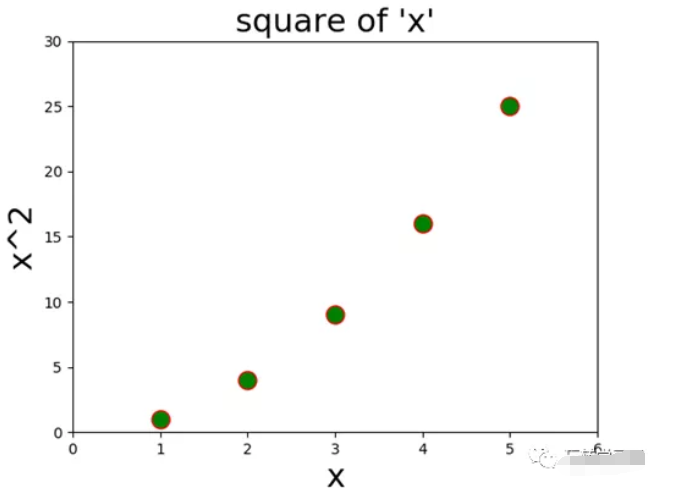解释:

（1）plt.scatter(xlist, ylist, edgecolor='r', c='g', s=150)，其中参数edgecolor表示边缘的颜色，此处设置为红色；参数c表示内部的颜色，此处设置为绿色；s表示点的大小；（注意：关于参数c还以如下设置c=(value1, value2, value3)，三个value取值范围0到1之间，分别表示红绿蓝三原色。plt.scatter(xlist,ylist, edgecolor='k',c=(1, 0, 0), s=150)见后面的图，注意区别）

（2）plt.axis([0, 6, 0, 30])作用设置横纵坐标轴的范围，前两个表示横坐标轴，后两个表示纵坐标轴。

import matplotlib.pyplot as plt
xlist = [1, 2, 3, 4, 5]
ylist = [x**2 for x in xlist]
plt.scatter(xlist, ylist, edgecolor='k',

c=(1, 0, 0), s=150)
plt.title("square of'x'", fontsize=22)
plt.xlabel("x", fontsize=22)
plt.ylabel("x^2", fontsize=22)
plt.axis([0, 6, 0, 30])
plt.tick_params(axis='both', labelsize=10)
plt.show()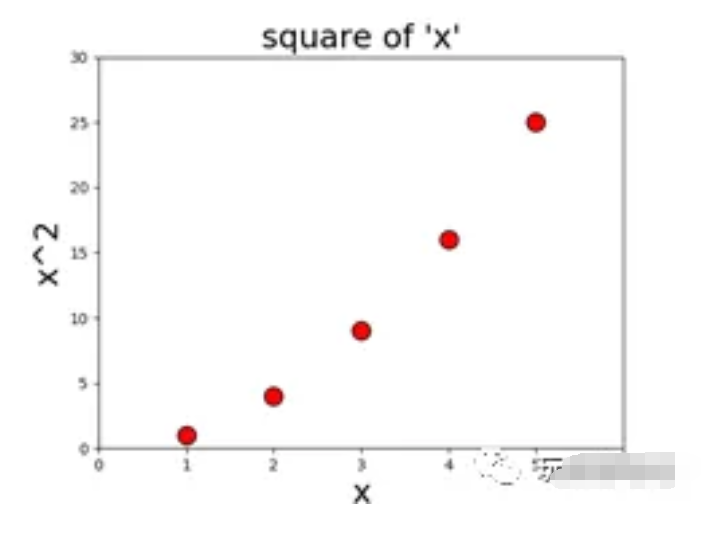全文图片：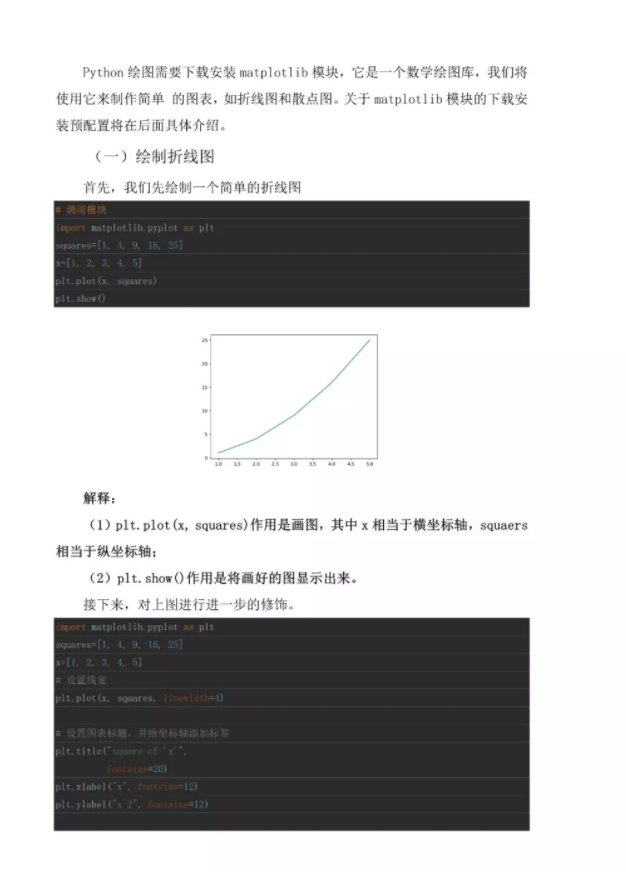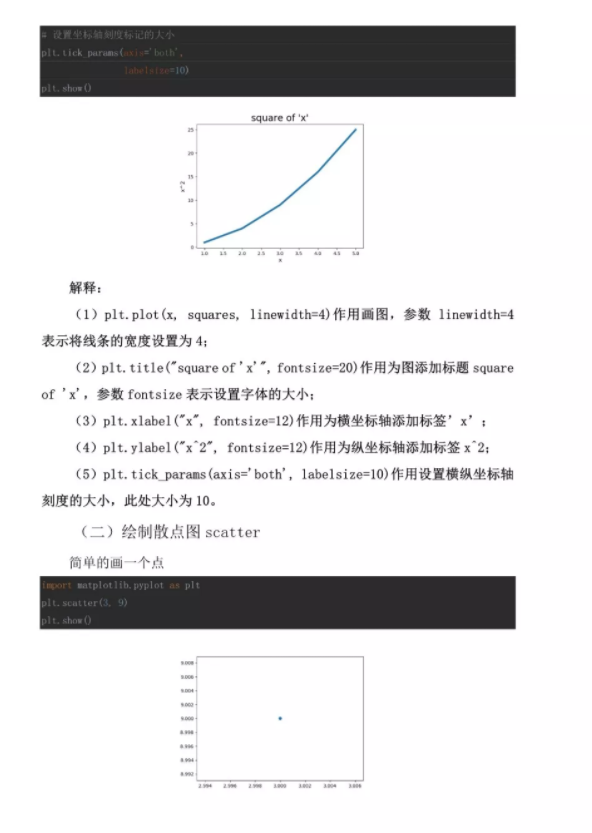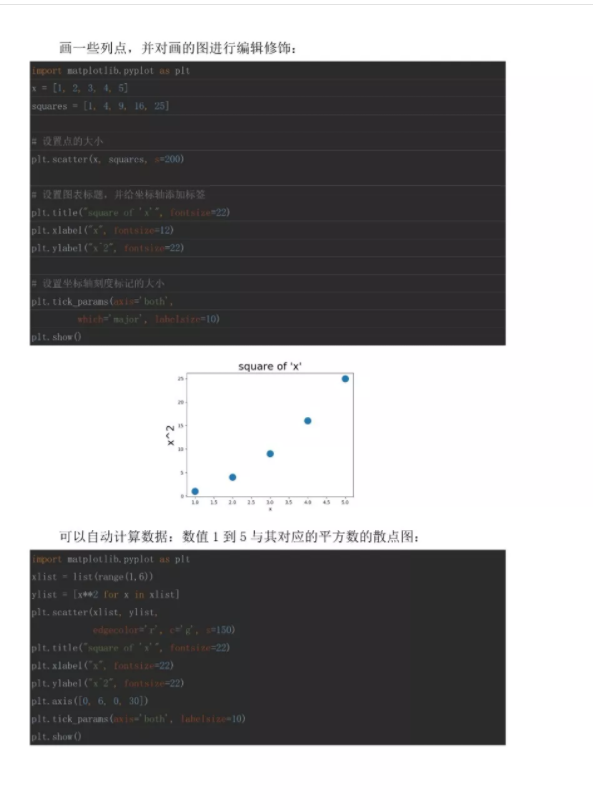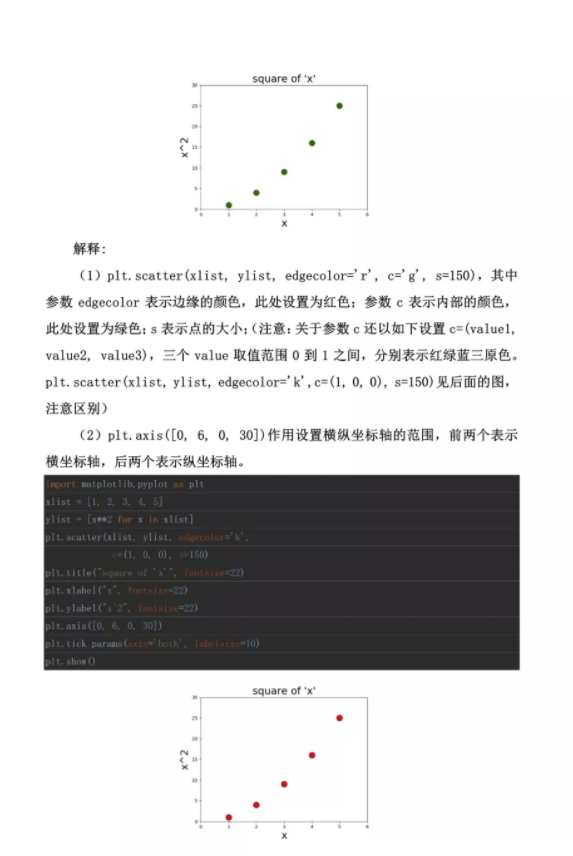展开全文大数据 机器学习
• 本篇文章给大家带来的内容是关于Python绘制折线图散点图的详细方法介绍（代码示例），有一定的参考价值，有需要的朋友可以参考一下，希望对你有所帮助。1、绘制折线图散点图要用到matplotlib下的pylab，因此我们...
本篇文章给大家带来的内容是关于Python绘制折线图和散点图的详细方法介绍（代码示例），有一定的参考价值，有需要的朋友可以参考一下，希望对你有所帮助。1、绘制折线图和散点图要用到matplotlib下的pylab，因此我们需要先引入，因为要用到数组实现，还要引入numpy。然后确定x轴和y轴的数据，最后将其呈现出来。import matplotlib.pylab as pylimport numpy as npx = [1, 3, 5, 6, 8, 13, 14, 16]y = [5, 1, 6, 7, 9, 3, 2, 10]pyl.plot(x, y)pyl.show()这样一个简单的折线图就绘制好了。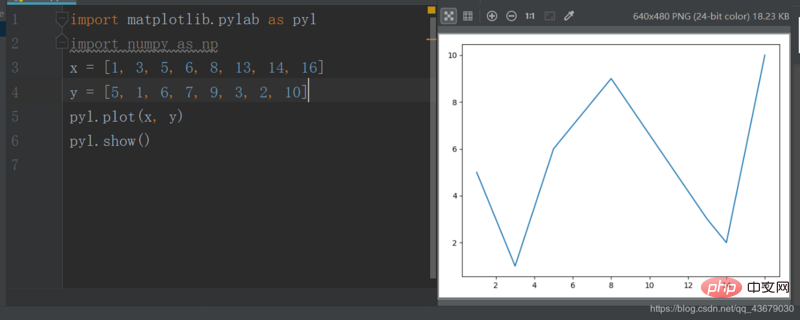上面的plot()有三个参数，第一个参数为x轴坐标，第二个参数为y轴坐标，第三个参数为确定线型，可有可无，如果要将上面的折线图改为散点图，只需更改第三个参数为"o’。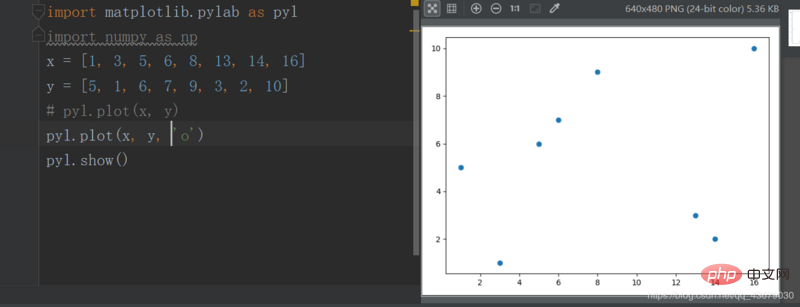如果让折线图和散点图叠加还可以突出每个点。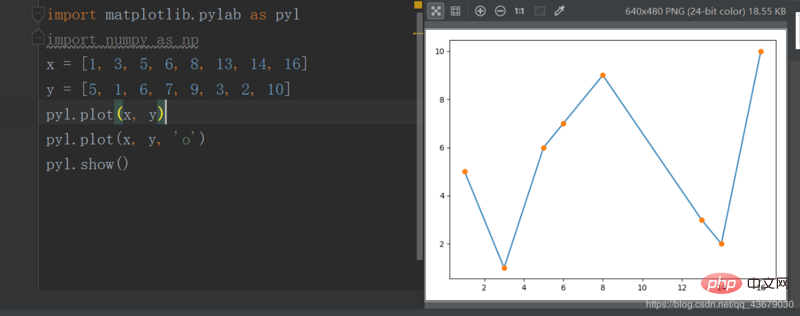2.我们还可以改变线和点的颜色，只需修改plot()的第三个参数。c--cyan--青色r--red--红色m--magente--品红g--green--绿色b--blue--蓝色y--yellow--黄色k--black--黑色w--white--白色上述参数可以叠加。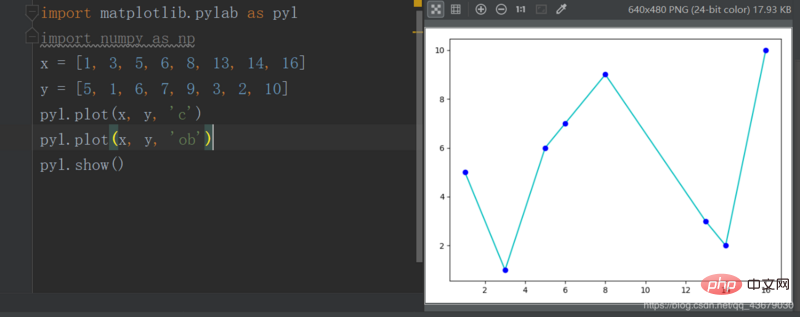3.我们还可以改变线型，也是修改plot的第三个参数。-   实线--    虚线-.    形式即为-.:    细小的虚线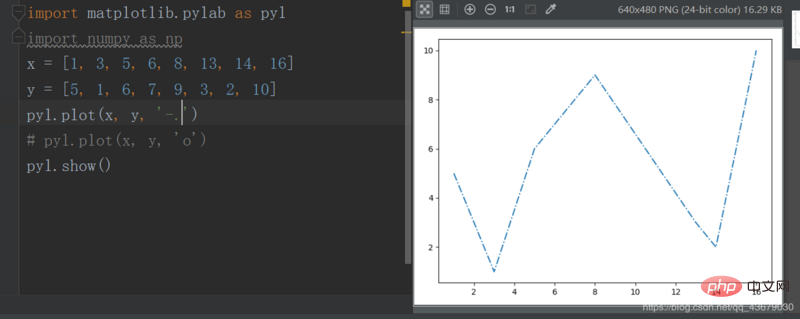4.我们还可以改变点型，同样是修改第三个参数。s--方形h--六角形H--六角形*--*形+--加号x--x形d--菱形D--菱形p--五角形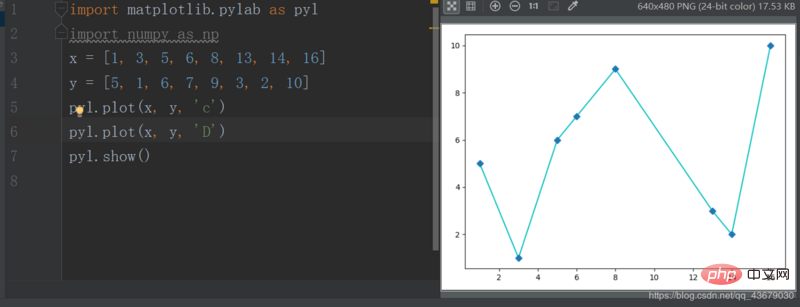5.我们目前绘制的图形，无图像名称及横纵坐标轴的名称，我们需要在程序中添加如下语句pyl.title()pyl.xlabel()pyl.ylabel()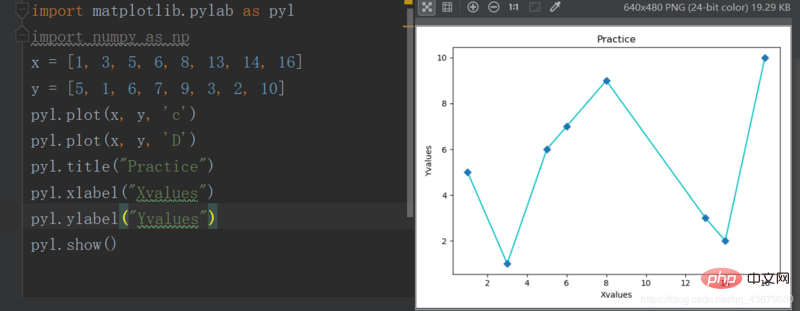6.现在绘图的x,y轴的范围是系统自动生成的，我们要想自定义，需要加上下面的两条语句，括号内为取值范围pyl.xlim()pyl.ylim()7.如果要在同一幅图中绘制多个图像，只需在show()之前再定义另外两个变量即可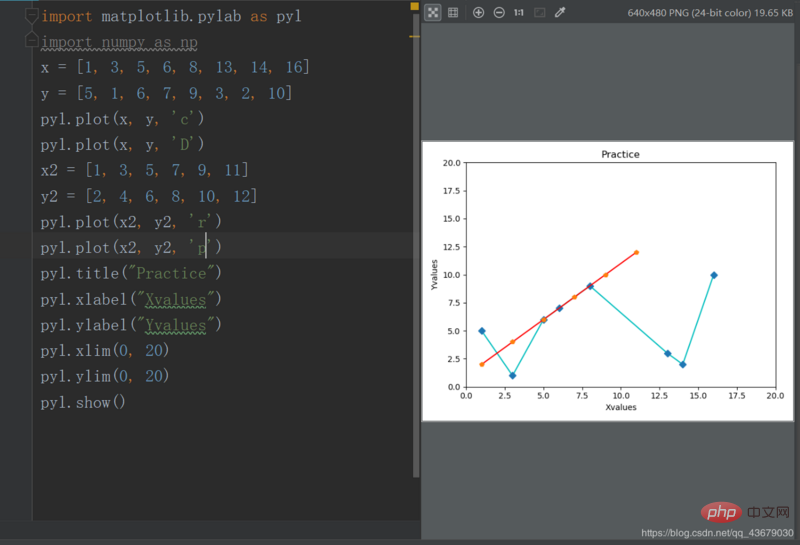以上就是Python绘制折线图和散点图的详细方法介绍（代码示例）的详细内容，更多请关注php中文网其它相关文章！本文转载于：segmentfault，如有侵犯，请联系a@php.cn删除
展开全文• 前提：画图必须要有数据，散点图数据自己构造的，折线图数据是通过线性回归模型预测的 from sklearn.linear_model import LinearRegression import matplotlib.pyplot as plt x = [[5.1], [3.5], [7.1], [6.2], ...
前提：画图必须要有数据，散点图数据自己构造的，折线图数据是通过线性回归模型预测的
from sklearn.linear_model import LinearRegression
import matplotlib.pyplot as plt

x = [[5.1], [3.5], [7.1], [6.2], [8.8], [7.8], [4.5], [5.6], [8.0], [6.4]]
y = [[1907.], [1287.], [2700.], [2373.], [3260.], [3000.], [1947.], [2273.], [3113.], [2493.]]

plt.scatter(x, y, label = 'scatter') #画散点图

linreg = LinearRegression()
linreg.fit(x, y)  # 回归拟合
y_pred = linreg.predict(x) #将袁术数据输入模型得到预测值

plt.plot(x, y_pred, 'r', label = 'plot')  #画折线图
plt.legend()
plt.show()
原始数据x与y变量存在线性关系，通过线性回归模型拟合两者的关系
y = 140 + 364 * x
140和364是模型拟和算出的，可以通过下面语句打印出来
print(linreg.intercept_)
print(linreg.coef_)
然后将x 输入到这个模型中，得到输出
[[1998.28164405]
[1415.59050279]
[2726.64557063]
[2398.88180367]
[3345.75490822]
[2981.57294493]
[1779.77246608]
[2180.3726257 ]
[3054.40933759]
[2471.71819633]]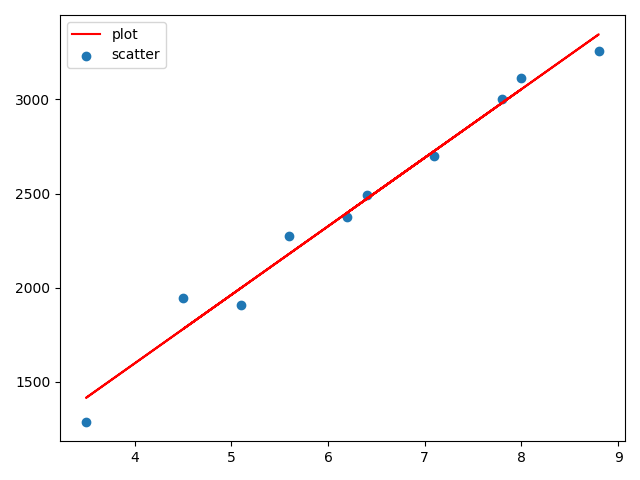展开全文matplotlib 线性回归
• python散点图 Python-散点图 (Python - Scatter Plots) Advertisements 广告 Previous Page 上一页 Next Page 下一页 Scatterplots show many points plotted in the Cartesian plane. Each point ...
• Python-画图（散点图scatter、保存savefig）及颜色大全 import numpy as np import matplotlib.pyplot as plt plt.rcParams['font.sans-serif']=['SimHei'] plt.rcParams['axes.unicode_minus'] = False #...
• 主要介绍了Python散点图折线图绘制过程解析,文中通过示例代码介绍的非常详细，对大家的学习或者工作具有一定的参考学习价值,需要的朋友可以参考下
• 找了篇教程学习matplotlib,写折线图散点图。首先pip install matplotlib安装。输入代码：import matplotlib.pyplot as pltinput_values =[1,2,3,4,5]squares = [1,4,9,16,25]#plt.plot(squares,linewidth=5)plt....
• #散点图，一般和相关分析、回归分析结合使用 import pandas import matplotlib import matplotlib.pyplot as plt plot_circle=pandas.read_csv('D://Python projects//reference data//6.1//data.csv') #定义主题...
• 散点图可以直观的查看数据的分布情况。 matplotlib模块的pyplot有画散点图的函数，但是该函数要求x轴是数字类型。 pandas的plot函数里，散点图类型scatter也要求数字型的，用时间类型的会报错。 在搜索阅读了几十...
• 本代码是利用python绘制散点图，使用xlrd读取Excel文件，
• 环境：windows系统，anaconda3 64位，python 3.61.初认识基本代码如下：import numpy as npimport matplotlib.pyplot as pltN = 1000x = np.random.randn(N)y = np.random.randn(N)plt.scatter(x, y)plt.show()这里...
• plt.scatter绘制散点图 plt.plot绘制折线图 使用scipy库可以进行曲线的smooth import matplotlib.pyplot as plt import numpy as np from scipy.interpolate import spline x = np.array([1, 3, 5, 7, 8]) y =...matplotlib 教学
• python绘制散点图标签：#Python##散点图#时间：2019/03/27 21:13:00作者：夏天的风今天下午学习了如何使用python绘制简单的散点图，写成博客分享一下。在python中画散点图主要是用matplotlib模块中的scatter函数，...
• 示例：散点图 最常见的散点图之一是x-y散点图。下面的代码会大致告诉你一个matplotlib是如何工作的，你会看到如何一点点建立起一个散点图。 我们正在使用点的x和y位置的一些构成数据。运行下面的代码，然后我们将...
• Python散点地图leftlet给R语言提供了很好用的交互式动态地图接口，其在Python中得API接口包名为folium（不知道包作者为何这样起名字，和leaflet已经扯不上关系了），可以满足我们平时常用的热力、填充地图、路径...
• python极坐标动态散点图 import numpy as np import matplotlib.pyplot as plt from matplotlib.animation import FuncAnimation # Data: beta = np.linspace(0.0 ,0.5,25) fig = plt.figure(figsize=(6,6)) ax......

# python动态散点图python 订阅